# Alternating algorithm

Jump to: navigation, search

An algorithm first proposed by J. von Neumann in 1933 [a6]. It gives a method for calculating the orthogonal projection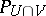onto the intersection of two closed subspaces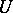andin a Hilbert space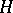in terms of the orthogonal projectionsand(cf. also Orthogonal projector). The result is that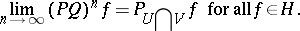It can be equivalently formulated as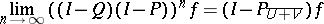(a1)

for all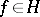. Here,is the orthogonal projection ofonto the subspace. Since it was first proposed, this algorithm has undergone many generalizations, mainly concerning the kind of spaces in which the algorithm can be located. It occurs in a large number of practical applications, such as domain decomposition methods for solving linear systems of equations, and certain multi-grid schemes used for solving elliptic partial differential equations. For a survey account of a wide collection of applications, see [a2].

The algorithm easily admits a generalization to a finite number of subspaces of. Letbe a member of the Hilbert space, and let,, be closed subspaces of. Let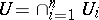, and let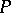be the orthogonal projection ofonto. Let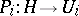be the orthogonal projection onto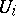,. Given, defineby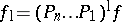, for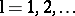. The elementsare the iterates in the alternating algorithm and the analogous convergence result is that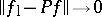as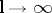. Quite a while later, other authors became interested in the rate of convergence of this algorithm. It was verified by N. Aronszain [a1] in the case of only two subspaces that the rate of convergence is usually linear. That is, there is a constantsuch that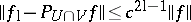for allin. The numberdepends on the notion of an angle between two subspaces of a Hilbert space. The anglebetweenandis given by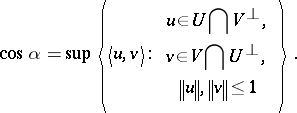In the present context,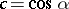. In [a7], K.T. Smith, D.C. Solman and S.L. Wagner gave the analogous result for more than two subspaces. The convergence constantremains a function of the angles between the subspaces, but is only a little more complicated than the case of two subspaces. Because of the importance of the rate of convergence, later authors have given improved rates. To get a very good expression for the rate of convergence, one needs to be very much more careful over the angles specified in the Smith–Solman–Wagner result. The best result know today (2000) is given in [a5].

The Lorch theorem states that the angle between two subspacesandof a Hilbert spaceis strictly positive if and only if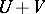is a closed subspace of. So if the sum is not closed, the result of Aronszain does not provide linear convergence, and it is of interest to enquire what takes place in this situation. A result of C. Franchetti and W.A. Light [a4] shows that ifis not closed, then the rate of convergence of the alternating algorithm may be arbitrarily slow.

Interesting questions arise when the algorithm is considered not as an algorithm in a Hilbert space, but in a Banach space with some fairly strong assumptions on the norm. The operators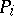andare then of course the operators of best approximation, so one needs at least to guarantee that such operators exist. For example, the ambient spacecan be a strictly convex and reflexive Banach space (cf. also Reflexive space; Smooth space). In fact, W.J. Stiles proved [a8] that if the dimension of such a space is at least, the convergence of the algorithm for allinand for all pairsandof closed subspaces suffices to guarantee thatis a Hilbert space.

Stiles also proved that in a finite-dimensional, smooth and strictly convex space, the alternating algorithm as given in (a1) is always effective. Several authors have given improvements to this results (see [a2]). The results of Franchetti and Light [a3] show that the convergence of the algorithm can still be linear in this case, with an appropriate modification to the definition of the angle between subspaces.

Finally, there are a number of interesting extensions where the projections are not uniquely defined. Instead, one may work in a spacewith subspacesandwhich do not guarantee unique best approximations. An example of this is the Diliberto–Straus algorithm, where,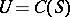and. In this algorithm, which is a realization of (a1), a selection must be employed to define the mappingsand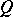.